## 0a. Intro

Macrophages were derived from freshly isolated bone marrow from mice of various genotypes. These cells were then infected with WNV-Tx i.c. p3 at an MOI of 2.5 for 24 or 48 hours (plus a 48h mock). RNA was then isolated and sent to Expression Analysis, Inc. The targets file of the analysis script describes the replication strategy and layout of all samples. The next steps are differential expression analysis to determine gene signatures specifically induced (or lacking) in each knockout. This script creates figures of interest from the differential gene expression results.

## 1. Set Locations

``````# Input files

in.expression <- "results/ExpressMatrix.txt"

# Input files for pathway annotation

in.immune <- "raw_data/GO_categories/GO0006955_immune_response.csv"

in.innate <- "raw_data/GO_categories/GO0045087_innate_immune_response.csv"

in.isgs <- "raw_data/ISGs_BMM_AH.csv"

in.th1 <- "raw_data/GO_categories/GO0042088_Th1_type_immune_response.txt"

in.th2 <- "raw_data/GO_categories/GO0042092_Th2_type_immune_response.txt"

in.m1 <- "raw_data/GO_categories/M1_Becker.txt"

in.m2 <- "raw_data/GO_categories/M2_Becker.txt"``````

## 2. Load Libraries and supplemental files

``````# Install libraries if needed

#source("http://www.bioconductor.org/biocLite.R")

#biocLite(pkgs = c("biomaRt", "xlsx", "limma", "plotrix", "reshape2", "ggplot2"))

library(biomaRt)

library(xlsx)``````
``## Loading required package: rJava``
``## Loading required package: xlsxjars``
``````library(limma)

library(plotrix)

library(reshape2)

library(ggplot2)

# Source in heatmap.F.4 function

source("../../MiscScripts/heatmap.F.R")``````
``````##

## Attaching package: 'gplots'``````
``````## The following object is masked from 'package:plotrix':

##

##     plotCI``````
``````## The following object is masked from 'package:stats':

##

##     lowess``````
``## Loading required package: fastcluster``
``````##

## Attaching package: 'fastcluster'``````
``````## The following object is masked from 'package:stats':

##

##     hclust``````
``## ``
``````## ==========================================================================

## *

## *  Package WGCNA 1.51 loaded.

## *

## *    but it is not enabled within WGCNA in R.

## *    To allow multi-threading within WGCNA with all available cores, use

## *

## *

## *    Alternatively, set the following environment variable on your system:

## *

## *

## *    for example

## *

## *

## *    To set the environment variable in linux bash shell, type

## *

## *

## *     before running R. Other operating systems or shells will

## *     have a similar command to achieve the same aim.

## *

## ==========================================================================``````
``````##

## Attaching package: 'WGCNA'``````
``````## The following object is masked from 'package:stats':

##

##     cor``````
``````# Print out session data

sessionInfo()``````
``````## R version 3.3.2 (2016-10-31)

## Platform: x86_64-w64-mingw32/x64 (64-bit)

## Running under: Windows 7 x64 (build 7601) Service Pack 1

##

## locale:

##  LC_COLLATE=English_United States.1252

##  LC_CTYPE=English_United States.1252

##  LC_MONETARY=English_United States.1252

##  LC_NUMERIC=C

##  LC_TIME=English_United States.1252

##

## attached base packages:

##  stats     graphics  grDevices utils     datasets  methods   base

##

## other attached packages:

##   WGCNA_1.51            fastcluster_1.1.22    dynamicTreeCut_1.63-1

##   gplots_3.0.1          ggplot2_2.2.1         reshape2_1.4.2

##   plotrix_3.6-4         limma_3.30.10         xlsx_0.5.7

##  xlsxjars_0.6.1        rJava_0.9-8           biomaRt_2.30.0

##

## loaded via a namespace (and not attached):

##   Rcpp_0.12.9           lattice_0.20-34       GO.db_3.4.0

##   gtools_3.5.0          assertthat_0.1        rprojroot_1.2

##   digest_0.6.12         foreach_1.4.3         plyr_1.8.4

##  backports_1.0.5       acepack_1.4.1         stats4_3.3.2

##  RSQLite_1.1-2         evaluate_0.10         lazyeval_0.2.0

##  data.table_1.10.4     gdata_2.17.0          S4Vectors_0.12.1

##  rpart_4.1-10          Matrix_1.2-8          preprocessCore_1.36.0

##  checkmate_1.8.2       rmarkdown_1.3         splines_3.3.2

##  stringr_1.1.0         foreign_0.8-67        htmlwidgets_0.8

##  RCurl_1.95-4.8        munsell_0.4.3         BiocGenerics_0.20.0

##  base64enc_0.1-3       htmltools_0.3.5       nnet_7.3-12

##  htmlTable_1.9         tibble_1.2            gridExtra_2.2.1

##  Hmisc_4.0-2           IRanges_2.8.1         codetools_0.2-15

##  matrixStats_0.51.0    XML_3.98-1.5          bitops_1.0-6

##  grid_3.3.2            gtable_0.2.0          DBI_0.5-1

##  magrittr_1.5          scales_0.4.1          KernSmooth_2.23-15

##  impute_1.48.0         stringi_1.1.2         doParallel_1.0.10

##  latticeExtra_0.6-28   Formula_1.2-1         RColorBrewer_1.1-2

##  iterators_1.0.8       tools_3.3.2           Biobase_2.34.0

##  parallel_3.3.2        survival_2.40-1       yaml_2.1.14

##  AnnotationDbi_1.36.2  colorspace_1.3-2      cluster_2.0.5

##  caTools_1.17.1        memoise_1.0.0         knitr_1.15.1``````

## 3. Create data structures needed for figure preparation

Read in differential expression matrix for union of differentially expressed genes

``````# DE = > 2 fold change up or down over mock, < 0.05 BH-adjusted p-val

# "masked" expression matrix: all non-significant genes set to 0

# Create DE results matrix from sigMask

DE_results <- apply(sigMask, MARGIN = c(1, 2), FUN = function(x) {

if (x >= 1) {

x = 1

} else if (x <= -1) {

x = -1

} else {

x = 0

}

})``````

Annotate genes according to ENSEMBL ID using biomaRt package

``````# Extract annotation from ENSEMBL database

ensembl <- useMart("ENSEMBL_MART_ENSEMBL", dataset = "mmusculus_gene_ensembl", host = "www.ensembl.org")

gene_map <- getBM(attributes = c("external_gene_name", "ensembl_gene_id"), filters = "external_gene_name",

values = row.names(expression), mart = ensembl)``````

Create function to collect heatmap modules

``````# gene_list is a named vector of genes mapped to respective modules

# exprs_matrix is matrix used to create heatmap

collect_modules <- function(gene_list, exprs_matrix) {

# Create filename for results (file named after expression matrix used to create heatmap)

filename <- paste("results/modules/", deparse(substitute(exprs_matrix)), ".xlsx", sep = "")

# Split vector of genes by module color

module_list <- split(gene_list, as.factor(gene_list))

# Collect the gene names mapping to each module color (vector names)

modules <- lapply(module_list, function(x) { names(x) })

# Collect relevant data for each module

module_expression <- lapply(modules, function(x) {

# collect annotation and expression data

annotation_data <- gene_map[(match(x, gene_map\$external_gene_name)), ]

express_data <- exprs_matrix[row.names(exprs_matrix) %in% x, ]

# reorder expression data to match annotation

express_data <- express_data[(match(x, row.names(express_data))), ]

cbind.data.frame(geneName = x, annotation_data\$ensembl_gene_id, express_data)

})

# Write results to file

for (i in 1:length(modules)) {

toAppend <- ifelse (i == 1, FALSE, TRUE)

module_color <- names(modules[i])

write.xlsx(module_expression[[i]], filename, sheetName = module_color, showNA = TRUE, col.names = TRUE,

row.names = FALSE, append = toAppend)

}

}``````

Create function to annotate gene list

``````# gene_list is a named vector of genes

# exprs_matrix is matrix of fold changes

annotate_gene_list <- function(gene_list, exprs_matrix) {

# collect annotation and expression data

annotation_data <- gene_map[(match(gene_list, gene_map\$external_gene_name)), ]

express_data <- exprs_matrix[row.names(exprs_matrix) %in% gene_list, ]

# reorder expression data to match annotation

express_data <- express_data[(match(gene_list, row.names(express_data))), ]

annotated <- cbind.data.frame(geneName = gene_list, ensembl = annotation_data\$ensembl_gene_id, express_data)

}``````

## 4. Heatmap of differentially expressed genes in WNV infection

Visualize union of WT differentially expressed genes

``````# Number of differentially expressed genes in at least one sample

length(row.names(expression))``````
``##  6823``
``````# Create heatmap of expression profile

express_map <- heatmap.F.4(expression, cutoff = 3, distmethod = "euclidean",clustermethod = "ward.D",

symbreaks = TRUE, margins = c(10, 1), keytitle = "log2 Fold Change")``````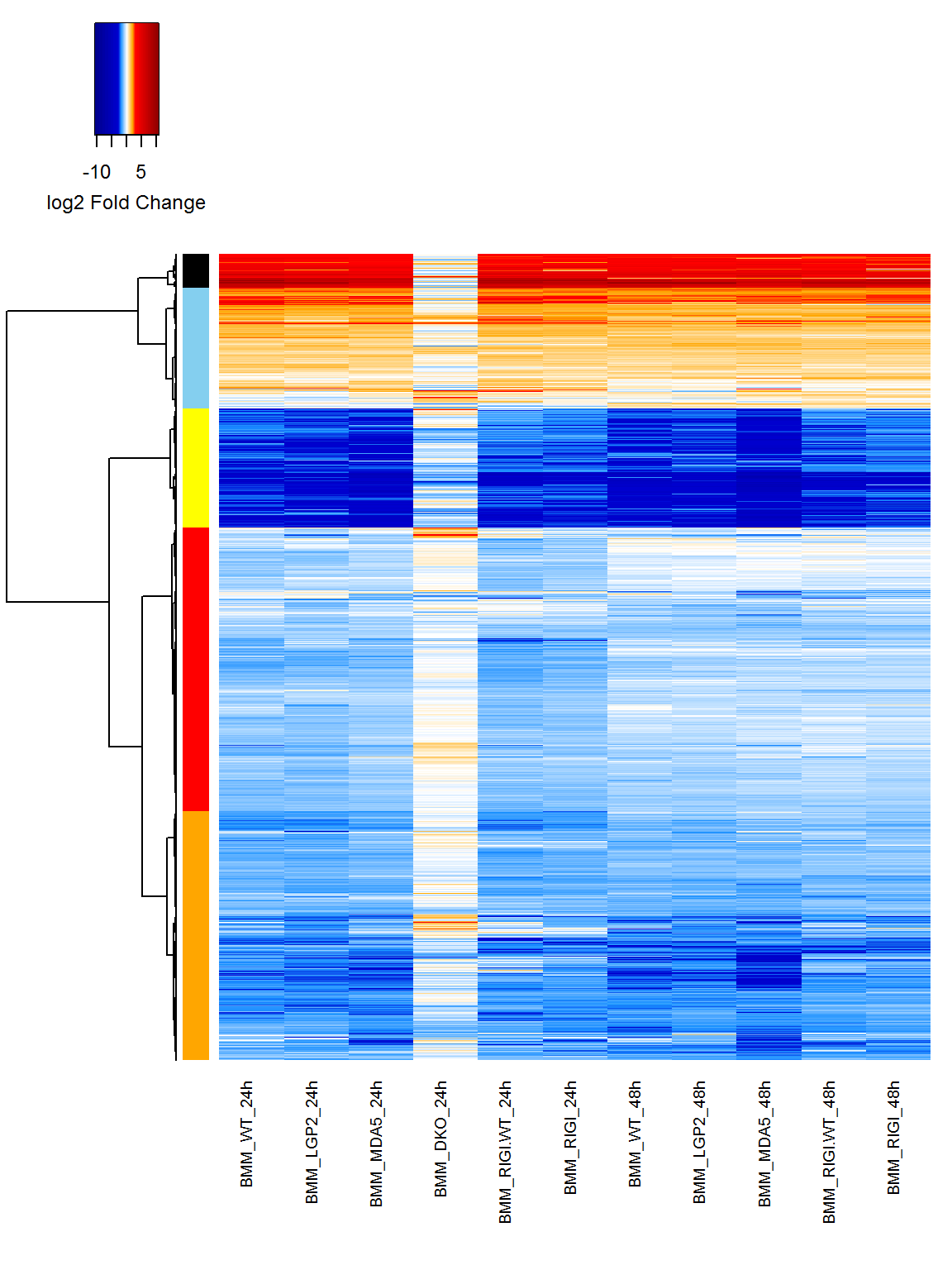``````# Write copy of heatmap to file

pdf("figures/Heatmap_DE_genes.pdf")

express_map <- heatmap.F.4(expression, cutoff = 3, distmethod = "euclidean", clustermethod = "ward.D",

symbreaks = TRUE, margins = c(10, 1), keytitle = "log2 Fold Change")

dev.off()``````
``````## png

##   2``````
``````# Write results of each module to file

collect_modules(express_map, expression)``````

Plot numbers of upregulated and downregulated genes

``````# Calculate numbers of upregulated and downregulated genes

updown <- t(apply(DE_results, MARGIN = 2, FUN = table))

updown <- as.data.frame(updown[, colnames(updown) != 0])

updown <- cbind(updown, sample = row.names(updown), ord = seq(1, length(row.names(updown))))

updown``````
``````##                   -1   1          sample ord

## BMM_WT_24h      3511 861      BMM_WT_24h   1

## BMM_LGP2_24h    3757 795    BMM_LGP2_24h   2

## BMM_MDA5_24h    3160 801    BMM_MDA5_24h   3

## BMM_DKO_24h      238 321     BMM_DKO_24h   4

## BMM_RIGI.WT_24h 3562 922 BMM_RIGI.WT_24h   5

## BMM_RIGI_24h    3350 840    BMM_RIGI_24h   6

## BMM_WT_48h      2783 759      BMM_WT_48h   7

## BMM_LGP2_48h    2694 762    BMM_LGP2_48h   8

## BMM_MDA5_48h    2886 810    BMM_MDA5_48h   9

## BMM_RIGI.WT_48h 2160 731 BMM_RIGI.WT_48h  10

## BMM_RIGI_48h    2369 746    BMM_RIGI_48h  11``````
``````# Reshape data frame for ggplot2 requirements

updown <- melt(updown, id.vars = c("sample", "ord"), variable.name = "direction", value.name = "count")

for (i in 1:(length(row.names(updown))/2)) {

updown[i, 4] <- updown[i, 4] * -1

}

updown\$pos <- updown\$count >= 0

# Create Plot

DE_plot <- ggplot(updown, aes(x = reorder(sample, ord), y = count, fill = pos)) +

geom_bar(stat = "identity", pos = "identity") +

scale_fill_manual(values = c("#0000FF", "#FF0000"), guide = FALSE) +

ylab("Number of Differentially Expressed Genes") +

theme_bw() +

theme(panel.grid.major.x = element_blank(),

panel.grid.minor.x = element_blank(),

panel.grid.major.y = element_blank(),

panel.grid.minor.y = element_blank(),

axis.text.x = element_text(angle = 90, hjust = 1, vjust = 0.5),

axis.title.x = element_blank())

print(DE_plot)``````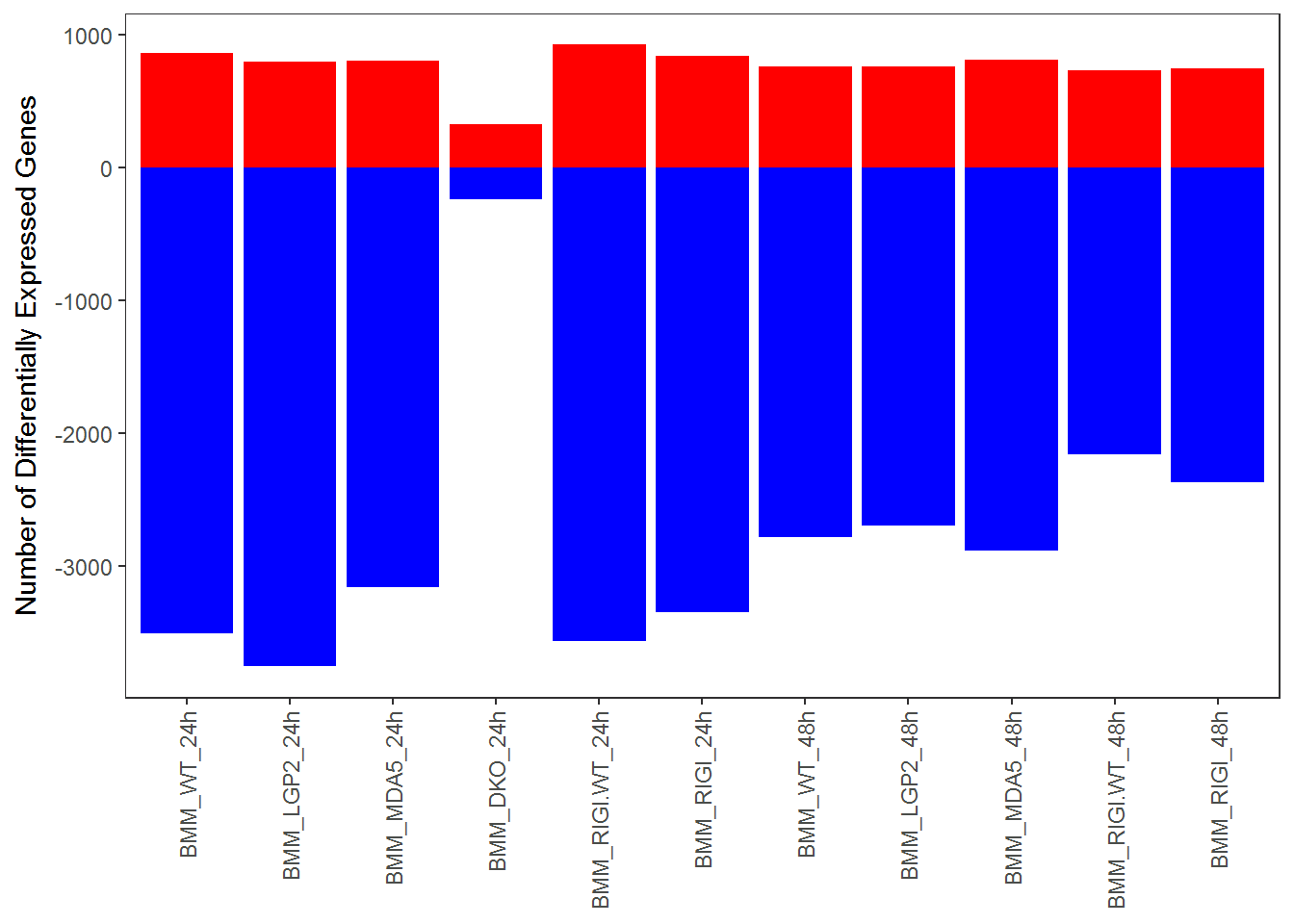``````# Create PDF of figure

pdf("figures/Barplot_DE_numbers.pdf")

print(DE_plot)

dev.off()``````
``````## png

##   2``````

## 5. Create Heatmaps of “wild-type” DE genes from Immune and Innate Immune Signaling GOBP categories

Immune response (GO:0006955)

``````# Read in gene list (single column)

# Extract DEs mapping to each category

immune_DEs <- expression[which(toupper(row.names(expression)) %in% immune), ]

# Number of differentially expressed immune genes

length(row.names(immune_DEs))``````
``##  532``
``````# Create heatmap of expression profile

immune_map <- heatmap.F.4(immune_DEs, cutoff = 3, distmethod = "euclidean", clustermethod = "ward.D",

symbreaks = TRUE, margins = c(10, 1), keytitle = "log2 Fold Change")``````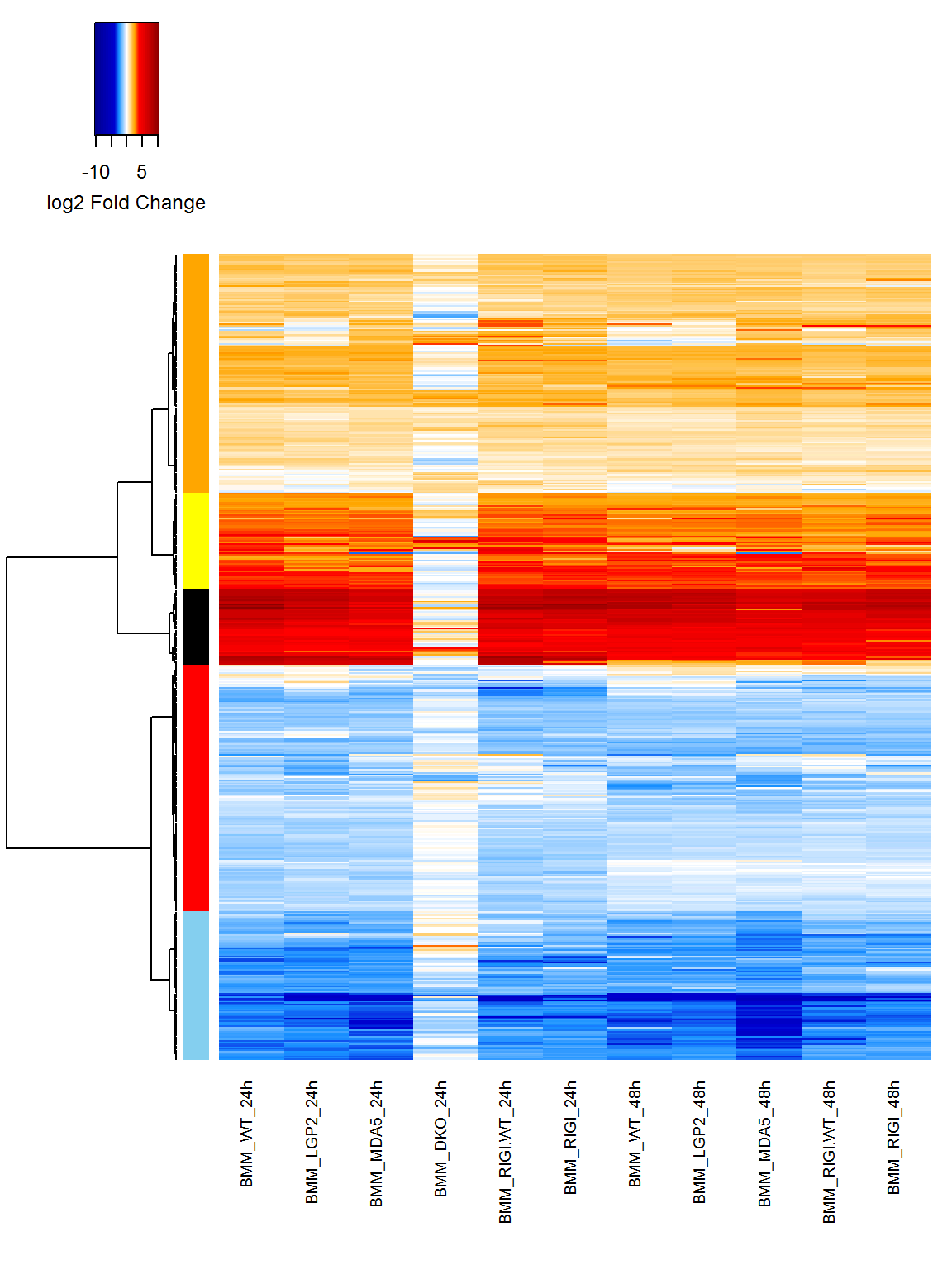``````# Create heatmaps from immune modules

pdf("figures/Heatmap_Immune_GO0006955_DE.pdf")

immune_map <- heatmap.F.4(immune_DEs, cutoff = 3, distmethod = "euclidean", clustermethod = "ward.D",

symbreaks = TRUE, margins = c(10, 1), keytitle = "log2 Fold Change")

dev.off()``````
``````## png

##   2``````
``````# Write results of each module to file

collect_modules(immune_map, immune_DEs)``````

Innate immune response (GO:0045087)

``````# Read in gene list (single column)

# Extract DEs mapping to each category

innate_DEs <- expression[which(toupper(row.names(expression)) %in% innate), ]

# Number of differentially expressed innate immune genes

length(row.names(innate_DEs))``````
``##  281``
``````# Create heatmap of expression profile

innate_map <- heatmap.F.4(innate_DEs, cutoff = 3, distmethod = "euclidean", clustermethod = "ward.D",

symbreaks = TRUE, margins = c(10, 1), keytitle = "log2 Fold Change")``````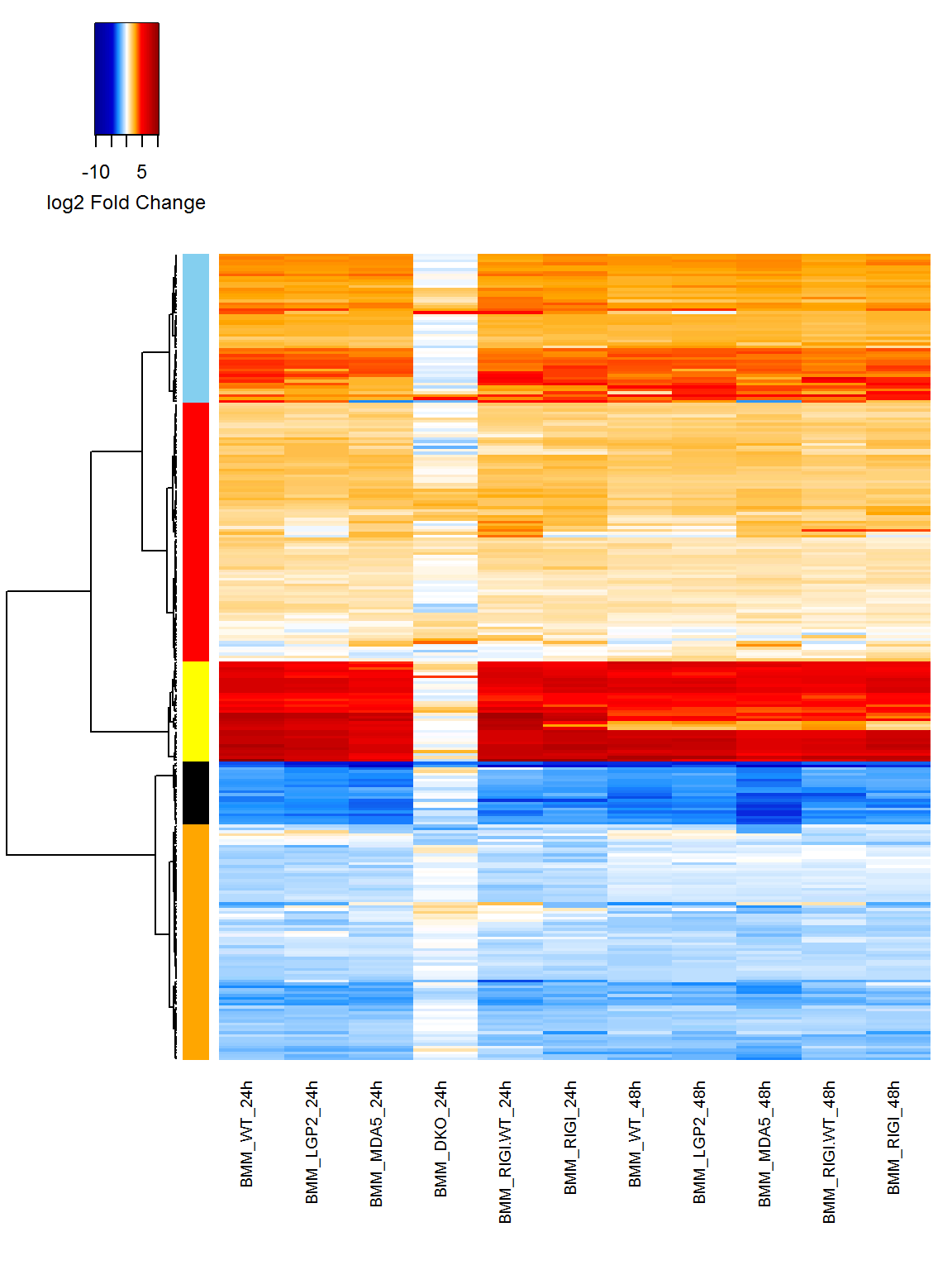``````# Create heatmaps from innate modules

pdf("figures/Heatmap_Innate_GO0045087_DE.pdf")

innate_map <- heatmap.F.4(innate_DEs, cutoff = 3, distmethod = "euclidean", clustermethod = "ward.D",

symbreaks = TRUE, margins = c(10, 1), keytitle = "log2 Fold Change")

dev.off()``````
``````## png

##   2``````
``````# Write results of each module to file

collect_modules(innate_map, innate_DEs)``````
1. Create heatmap of all WNV-induced ISGs among differentially expressed genes ISGs defined per IFN-beta treatment of BMM for 6 or 24 hours inducing > 2-fold gene induction (Al Huang data set)
``````# Read in measurement of IFN-beta treatment of BMM: 6 and 24 hours

# Which genes are induced > 2-fold at either 6 or 24hr post-IFN-beta treatment in BMM?

ISGs <- row.names(ISG_expression[which(ISG_expression["mac_beta_vs_mock_6h"] > 1 |

ISG_expression["mac_beta_vs_mock_24h"] > 1), ])

# Extract WNV DEs mapping to ISG list

ISG_DEs <- expression[which(toupper(row.names(expression)) %in% toupper(ISGs)), ]

# Number of differentially expressed ISGs

length(row.names(ISG_DEs))``````
``##  341``
``````# Create heatmap of expression profile

ISG_map <- heatmap.F.4(ISG_DEs, cutoff = 4, distmethod = "euclidean", clustermethod = "ward.D",

symbreaks = TRUE, margins = c(10, 1), keytitle = "log2 Fold Change")``````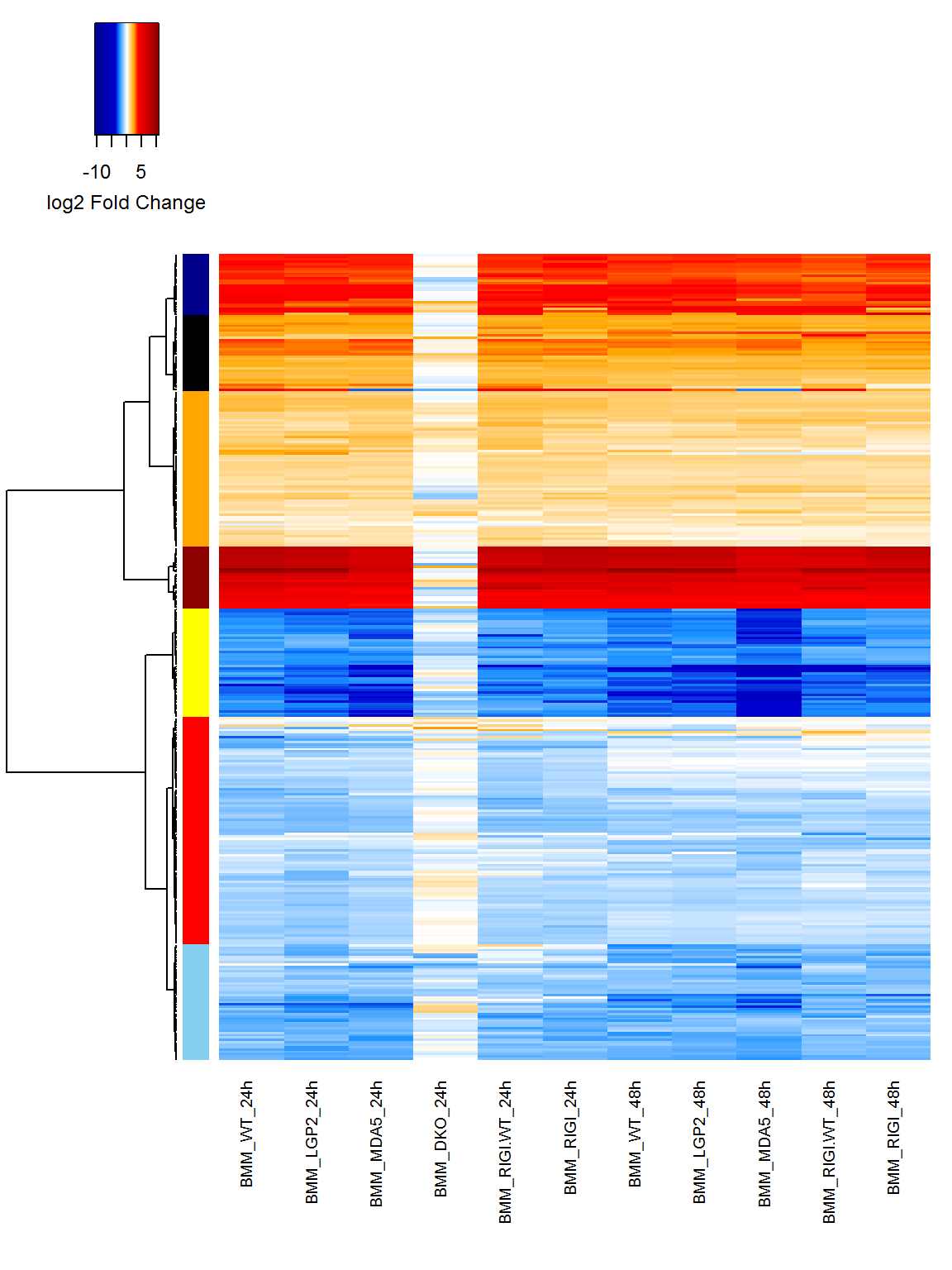``````# Create heatmaps from ISG lists

pdf("figures/Heatmap_ISG_DE.pdf")

ISG_map <- heatmap.F.4(ISG_DEs, cutoff = 4, distmethod = "euclidean", clustermethod = "ward.D",

symbreaks = TRUE, margins = c(10, 1), keytitle = "log2 Fold Change")

dev.off()``````
``````## png

##   2``````
``````# Write results of each module to file

collect_modules(ISG_map, ISG_DEs)``````

Non-ISGs differentially expressed following WNV infection (all genes NOT in above list)

``````# Extract WNV DEs NOT mapping to ISG list

non_ISG_DEs <- expression[-which(toupper(row.names(expression)) %in% toupper(ISGs)), ]

# Number of differentially expressed non-ISGs

length(row.names(non_ISG_DEs))``````
``##  6482``
``````# Create heatmap of expression profile

non_ISG_map <- heatmap.F.4(non_ISG_DEs, cutoff = 3, distmethod = "euclidean", clustermethod = "ward.D",

symbreaks = TRUE, margins = c(10, 1), keytitle = "log2 Fold Change")``````